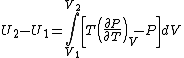# Isothermal Process

Also found in: Dictionary, Thesaurus, Medical, Wikipedia.
Related to Isothermal Process: Isentropic process

## Isothermal process

A thermodynamic process which occurs with a heat addition or removal rate just adequate to maintain constant temperature. The change in the internal energy per mole U accompanying a change in volume in an isothermal process is given by the equation below, where T is thetemperature, P is the pressure, and V is the volume per mole. The integral in the equation is zero for an ideal gas, and approximately zero for a condensed phase (solid or liquid) for which the volume changes vary little with pressure. Thus, in both these cases, U2 = U1. For real gases, the integral is nonzero, and the internal energy change is computed using the equation of state of the gas in the equation. See Thermodynamic processes

## Isothermal Process

a process that occurs in a physical system at constant temperature.

To create an isothermal process a system is usually placed in a thermostat (a massive body in thermoequilibrium) of high thermal conductivity, so that heat exchange with the system proceeds quite rapidly and the difference between the temperature of the system and that of the thermostat is negligible. Another method is to use heat sources or heat sinks while checking for constant temperature by means of thermometers. Boiling a fluid and melting a solid at constant pressure, for example, are isothermal processes. For an ideal gas, the product of pressure by volume is constant during an isothermal process (the Boyle-Mariotte law).

Generally speaking, a certain amount of heat is conveyed to (or given off from) the system in an isothermal process, and external work is performed. The work performed by an ideal gas in an isothermal process is equal to NkT log (V2/V1), where N is the number of particles of the gas, T is the temperature, and K2 are the volumes of the gas at the start and completion of the process, and k is Boltzmann’s constant.

In solids and in most liquids an isothermal process changes the volume of a body very little, as long as there is no phase transition.

V. L. POKROVSKII [10–291—4]

## isothermal process

[¦ī·sə¦thər·məl ′prä·səs]
(thermodynamics)
Any constant-temperature process, such as expansion or compression of a gas, accompanied by heat addition or removal from the system at a rate just adequate to maintain the constant temperature.
References in periodicals archive ?
Completing the investigation of the isothermal process of pressure solidification involves the evaluation of the procedure itself.
It is difficult, therefore, to estimate accurately the value of the crystallinity directly from the crystallization curve in the initial stage of the isothermal process, especially at low crystallization temperatures.
It is noteworthy that in the analysis of the crystallization kinetics, the cooling time has been previously subtracted and only the isothermal process has been considered, as it has been described in the rheological experimental section (points A and B).
The only way in which both Eqs 2 and 10 are jointly satisfied at all times for an isothermal process is when
Then, the Avrami model can be used to describe the development of the relative degree of crystallization in the isothermal process according to the following equation:

Site: Follow: Share:
Open / Close